# NCERT Solutions for Class 6 Maths Chapter 13 Symmetry (Ex 13.2) Exercise 13.2

## NCERT Solutions for Class 6 Maths Chapter 13 Symmetry (Ex 13.2) Exercise 13.2

Free PDF download of NCERT Solutions for Class 6 Maths Chapter 13 Exercise 13.2 (Ex 13.2) and all chapter exercises at one place prepared by an expert teacher as per NCERT (CBSE) books guidelines. Class 6 Maths Chapter 13 Symmetry Exercise 13.2 Questions with Solutions to help you to revise complete Syllabus and Score More marks. Register and get all exercise solutions in your emails.

Every NCERT Solution is provided to make the study simple and interesting on Vedantu. Subjects like Science, Maths, English,Hindi will become easy to study if you have access to NCERT Solution for Class 6 Science , Maths solutions and solutions of other subjects.

Do you need help with your Homework? Are you preparing for Exams?
Study without Internet (Offline)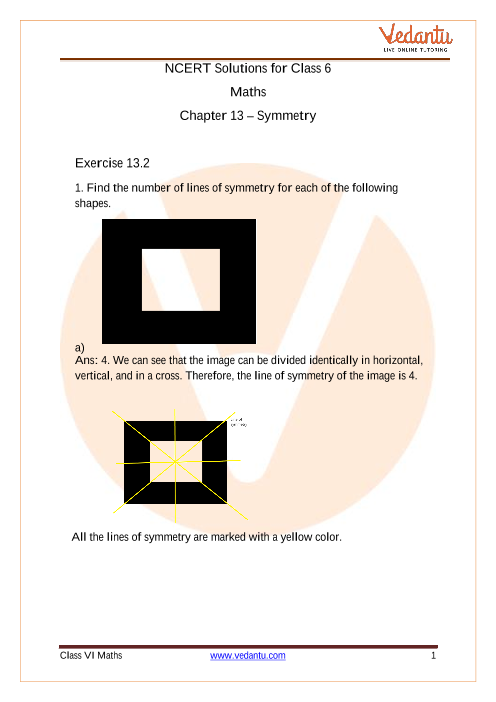Book your Free Demo session
Get a flavour of LIVE classes here at Vedantu## Access NCERT Solutions for Maths Class 6 Chapter 13 – Symmetry

Exercise 13.2

1. Find the number of lines of symmetry for each of the following shapes.

a)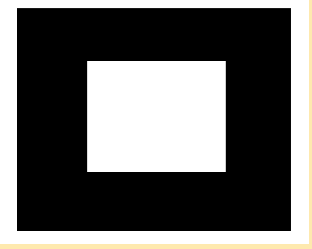Ans: 4. We can see that the image can be divided identically in horizontal, vertical, and in a cross. Therefore, the line of symmetry of the image is 4.

All the lines of symmetry are marked with a yellow colour.

b)Ans: 4. We can see that the image can be divided identically in horizontal, vertical, and in a cross. Therefore, the line of symmetry of the image is 4.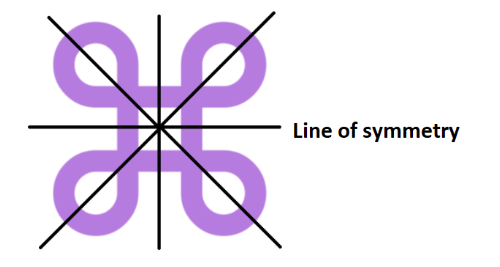c)Ans: 4. We can see that the image can be divided identically in horizontal, vertical, and in a cross. Therefore, the line of symmetry of the image is 4.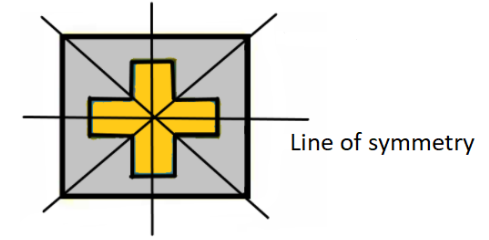d)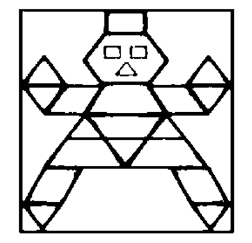Ans: 1. We can see that the image can be divided identically in horizontal, vertical, and in a cross. Therefore, the line of symmetry of the image is 1.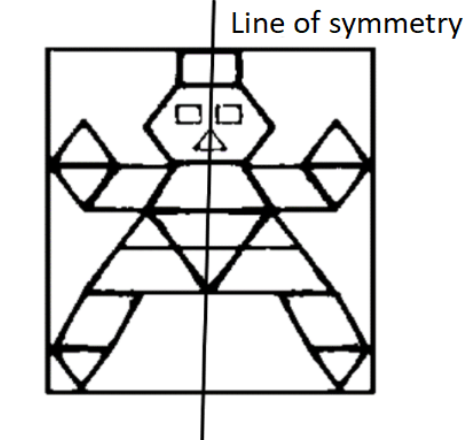e)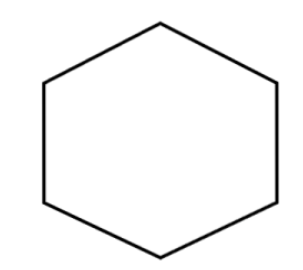Ans: 6. We can see that the image can be divided identically in horizontal, vertical, and in a cross. Therefore, the line of symmetry of the image is 6.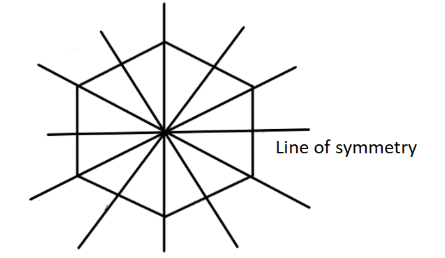f)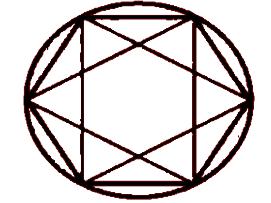Ans: 6. We can see that the image can be divided identically in horizontal, vertical, and in a cross. Therefore, the line of symmetry of the image is 6.

The lines of symmetry are made with yellow color.

g)

Ans: 0. We can see that the image can be divided identically in horizontal, vertical, and in a cross. Therefore, the line of symmetry of the image is 0.

h)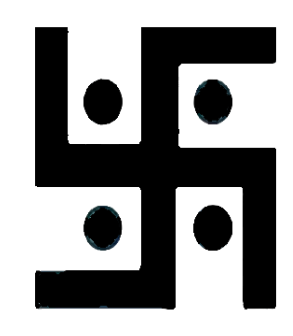Ans: 0. We can see that the image can be divided identically in horizontal, vertical, and in a cross. Therefore, the line of symmetry of the image is 0.

i)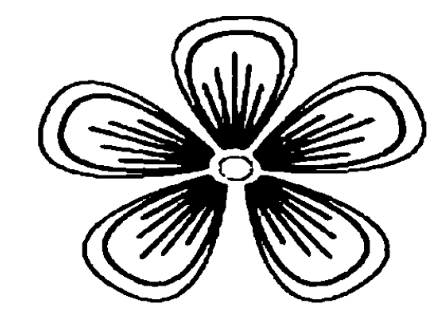Ans: 5. We can see that the image can be divided identically in horizontal, vertical, and in a cross. Therefore, the line of symmetry of the image is 5.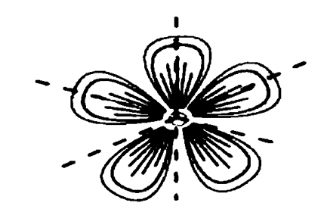2. Copy the following drawing on squared paper. Complete each one of them such that the resulting figure has two dotted lines as two lines of symmetry. How did you go about completing the picture?

a)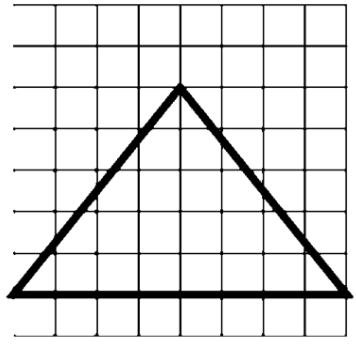Ans: The drawing is copied and drawn wisely such that the two dotted lines are now the line of symmetry of the drawing.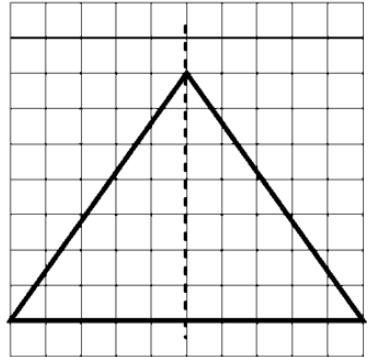b)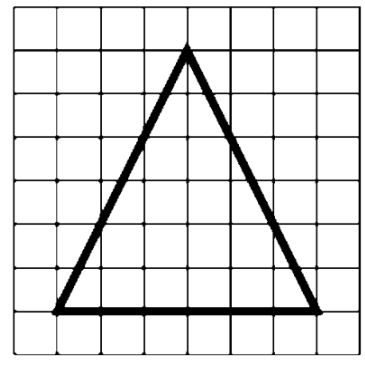Ans: The drawing is copied and drawn wisely such that the two dotted lines are now the line of symmetry of the drawing.c)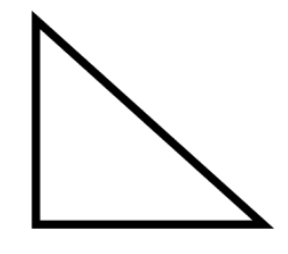Ans: The drawing is copied and drawn wisely such that the two dotted lines are now the line of symmetry of the drawing.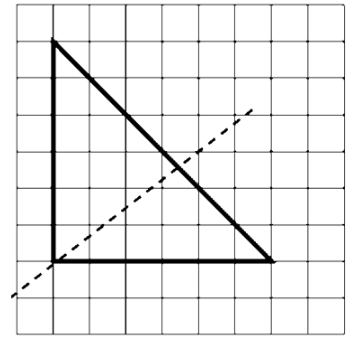d)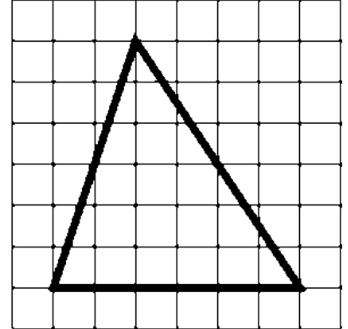Ans: The drawing is copied below. The given triangle is a scalene triangle so, it will not have any line of symmetry.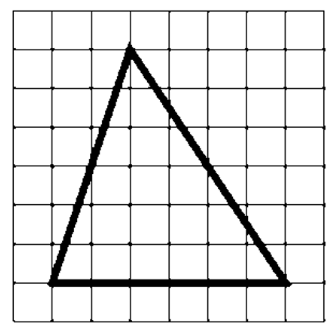3. Complete the following table.

 Shape Rough figure No. of lines of symmetry EquilateralTriangle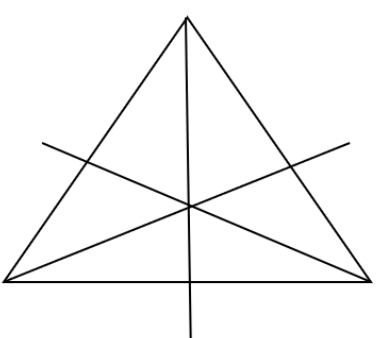3 Square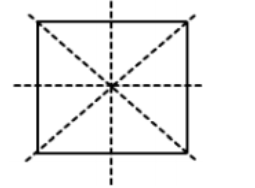4 Rectangle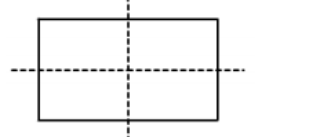2 IsoscelesTriangle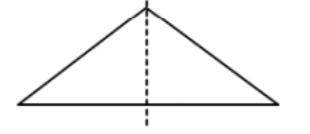1 Rhombus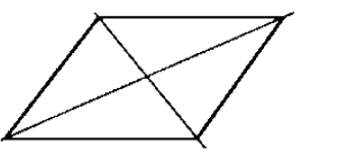2 Circle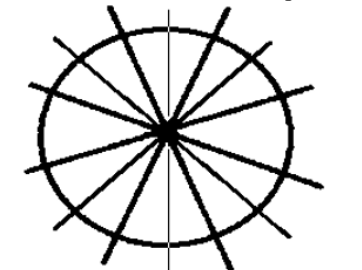Infinite

4. Can you draw a triangle which has:

a) Exactly one line of symmetry?

Ans: We can draw an isosceles triangle.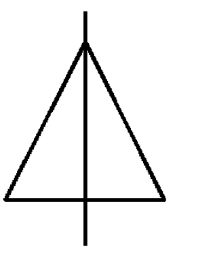b) Exactly two lines of symmetry?

Ans: It is impossible.

c) Exactly three lines of symmetry?

Ans: We can draw an equilateral triangle.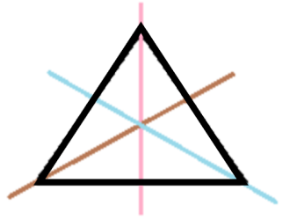d) No line of symmetry

Ans: We can draw a right angled triangle.5. On a squared paper, sketch the following

a) A triangle with a horizontal line of symmetry but no vertical line of symmetry.

Ans: A triangle with a horizontal line of symmetry but no vertical line of symmetry can be drawn in the following way.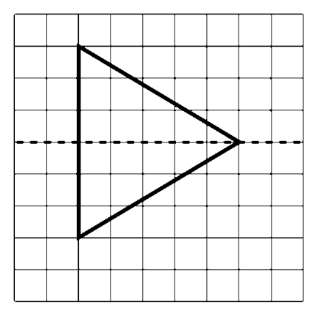b) A quadrilateral with both horizontal and vertical lines of symmetry

Ans: A quadrilateral with both horizontal and vertical lines of symmetry can be drawn in the following way.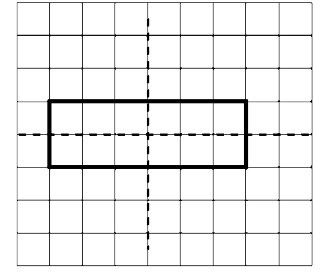c) A quadrilateral with both horizontal lines of symmetry but no vertical lines of symmetry

Ans: A quadrilateral with both horizontal lines of symmetry but no vertical lines of symmetry can be drawn in the following way.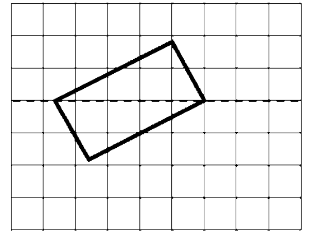d) A hexagon with exactly two lines of symmetry

Ans: A hexagon with exactly two lines of symmetry can be drawn in the following way.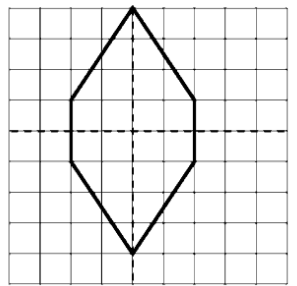e) A hexagon with six lines of symmetry.

Ans: A hexagon with six lines of symmetry is given below.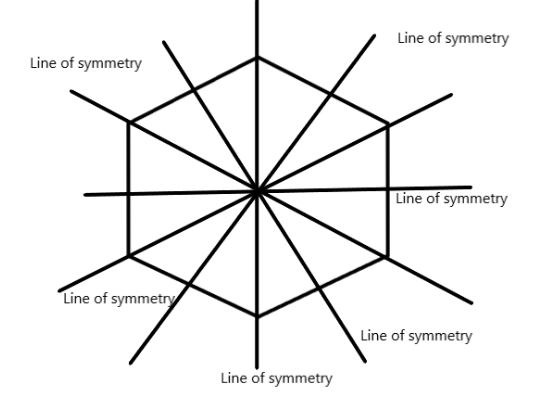6. Trace each figure and draw the lines of symmetry, if any:

a)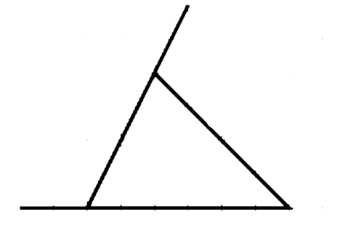Ans: Lines of symmetry are drawn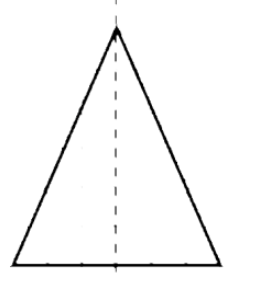b)

Ans: Lines of symmetry are drawn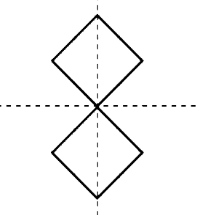c)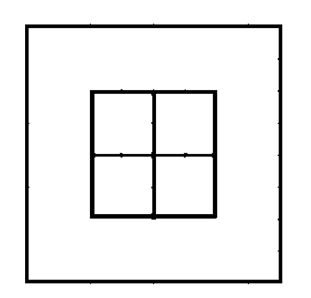Ans: Lines of symmetry are drawn.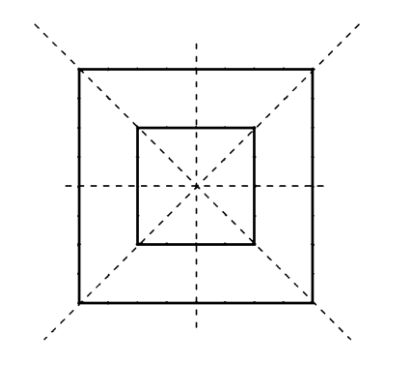d)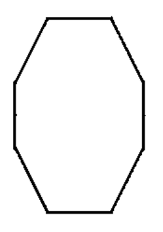Ans: Lines of symmetry are drawn.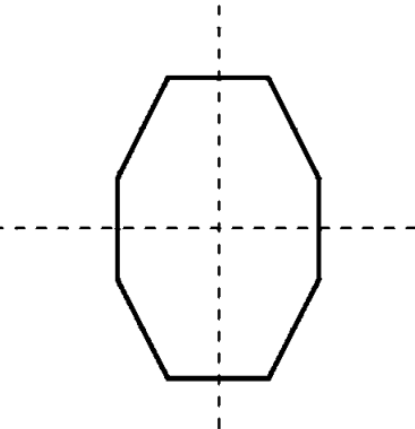e)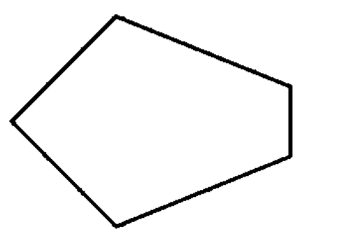Ans: Lines of symmetry are drawn.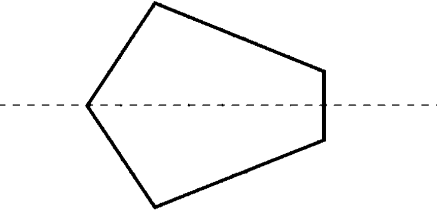f)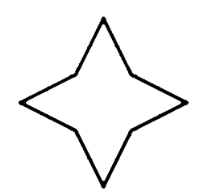Ans: Lines of symmetry are drawn.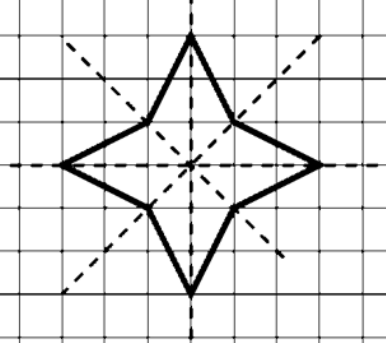7. Consider the letters of English alphabets A to Z. List among them the letters which have:

a) Vertical lines of symmetry ( like A )

Ans: A, H, I, M, O, T, U, V, W, X, Y

b) Horizontal lines of symmetry ( like B )

Ans: B, C, D, E, H, I, K, O, X

c) No lines of symmetry ( like Q )

Ans: F, G, J, L, N, P, Q, R, S, Z

8. Given here are figures of a few folded sheets and a design drawn about the fold. In each case, draw a rough diagram of the complete figure that would be seen when the design is cut off.

a)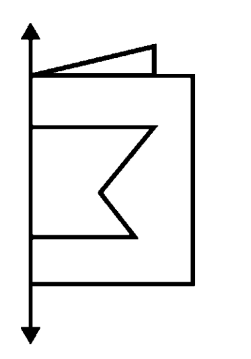Ans: A rough diagram of the complete figure is drawn.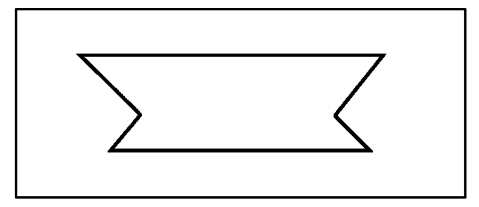b)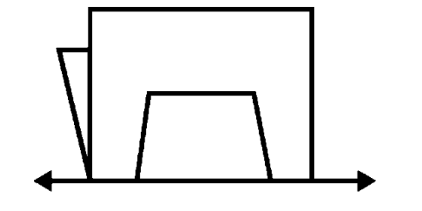Ans: A rough diagram of the complete figure is drawn.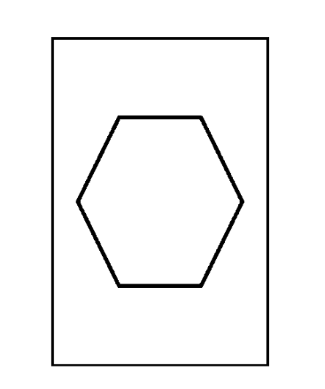## NCERT Solutions for Class 6 Maths Chapter 13 Symmetry Exercise 13.2

Opting for the NCERT solutions for Ex 13.2 Class 6 Maths is considered as the best option for the CBSE students when it comes to exam preparation. This chapter consists of many exercises. Out of which we have provided the Exercise 13.2 Class 6 Maths NCERT solutions on this page in PDF format. You can download this solution as per your convenience or you can study it directly from our website/ app online.

Vedantu in-house subject matter experts have solved the problems/ questions from the exercise with the utmost care and by following all the guidelines by CBSE. Class 6 students who are thorough with all the concepts from the Maths textbook and quite well-versed with all the problems from the exercises given in it, then any student can easily score the highest possible marks in the final exam. With the help of this Class 6 Maths Chapter 13 Exercise 13.2 solutions, students can easily understand the pattern of questions that can be asked in the exam from this chapter and also learn the marks weightage of the chapter. So that they can prepare themselves accordingly for the final exam.

Besides these NCERT solutions for Class 6 Maths Chapter 13 Exercise 13.2, there are plenty of exercises in this chapter which contain innumerable questions as well. All these questions are solved/answered by our in-house subject experts as mentioned earlier. Hence all of these are bound to be of superior quality and anyone can refer to these during the time of exam preparation. In order to score the best possible marks in the class, it is really important to understand all the concepts of the textbooks and solve the problems from the exercises given next to it.

Do not delay any more. Download the NCERT solutions for Class 6 Maths Chapter 13 Exercise 13.2 from Vedantu website now for better exam preparation. If you have the Vedantu app in your phone, you can download the same through the app as well. The best part of these solutions is these can be accessed both online and offline as well.SHARETWEETSHARESUBSCRIBE